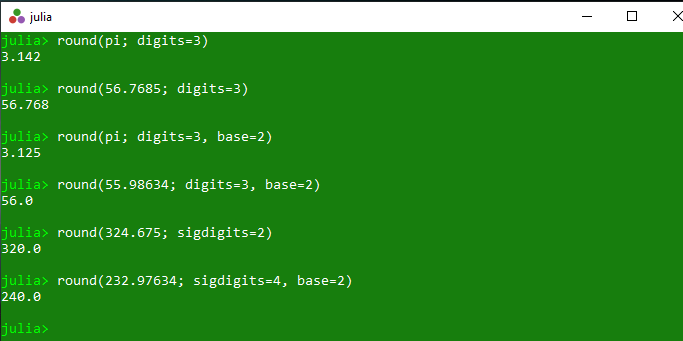# Getting rounded value of a number in Julia – round() Method

The `round()` is an inbuilt function in julia which is used to round the specified number in different ways which are illustrated below-

• The default round process is done to the nearest integer, with ties (fractional values of 0.5) being rounded to the nearest even integer.
• The specified value x is rounded to an integer value and returns a value of the given type T or of the same type of x.
• If the digits keyword parameter is given, it rounds to the specified number of digits after the decimal place (or before if negative), in the given base.
• If the sigdigits keyword parameter is given, it rounds to the specified number of significant digits, in the given base.

Syntax:
round(x)
round(T, x)
round(x, digits::Integer, base)
round(x, sigdigits::Integer, base)

Parameters:

• x: Specified number
• T: Specified number type
• digits: Specified digit values
• base: Specified base in which results are coming
• sigdigits: Specified significant digits

Returns: It returns the rounded value of the specified number.

Example 1:

 `# Julia program to illustrate  ` `# the use of round() method ` ` `  `# Getting the rounded values ` `println(``round``(``2``)) ` `println(``round``(``2.3``)) ` `println(``round``(``2.9``)) ` `println(``round``(``0.5``)) ` `println(``round``(``2.5``)) ` `println(``round``(``3.5``)) `

Output:

```2
2.0
3.0
0.0
2.0
4.0
```

Example 2:

 `# Julia program to illustrate  ` `# the use of round() method ` ` `  `# Getting the rounded values ` `println(``round``(pi; digits ``=` `3``)) ` `println(``round``(``56.7685``; digits ``=` `3``)) ` `println(``round``(pi; digits ``=` `3``, base ``=` `2``)) ` `println(``round``(``55.98634``; digits ``=` `3``, base ``=` `2``)) ` `println(``round``(``324.675``; sigdigits ``=` `2``)) ` `println(``round``(``232.97634``; sigdigits ``=` `4``, base ``=` `2``)) `

Output:My Personal Notes arrow_drop_upCheck out this Author's contributed articles.

If you like GeeksforGeeks and would like to contribute, you can also write an article using contribute.geeksforgeeks.org or mail your article to contribute@geeksforgeeks.org. See your article appearing on the GeeksforGeeks main page and help other Geeks.

Please Improve this article if you find anything incorrect by clicking on the "Improve Article" button below.

Article Tags :

Be the First to upvote.

Please write to us at contribute@geeksforgeeks.org to report any issue with the above content.